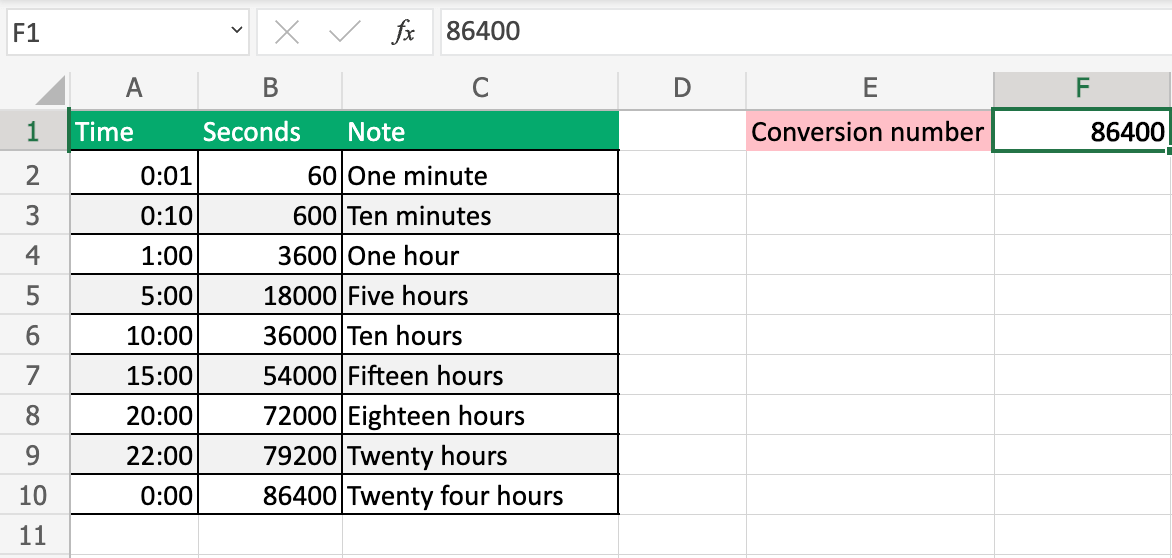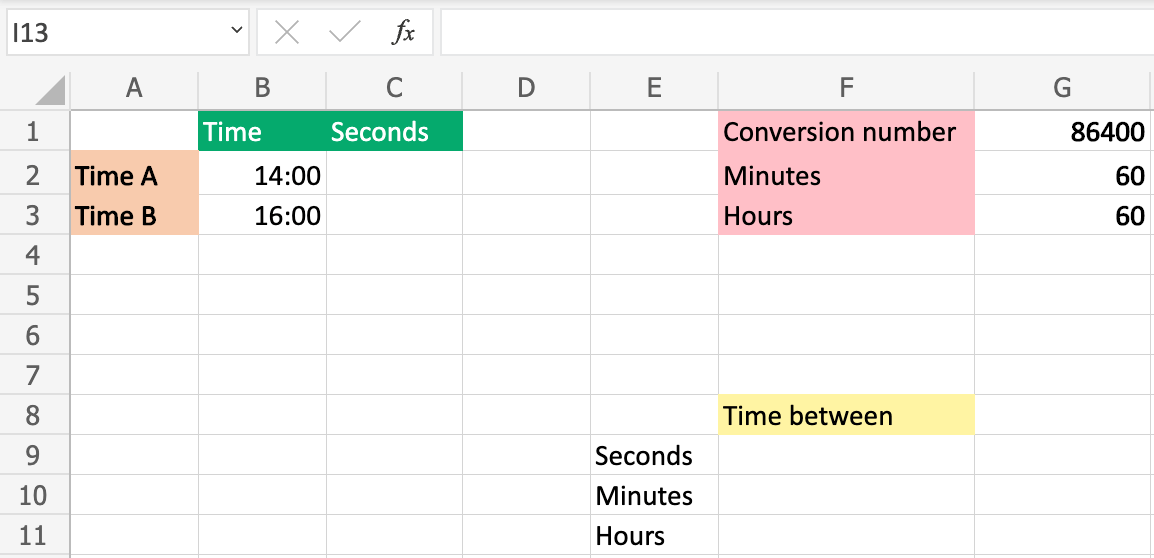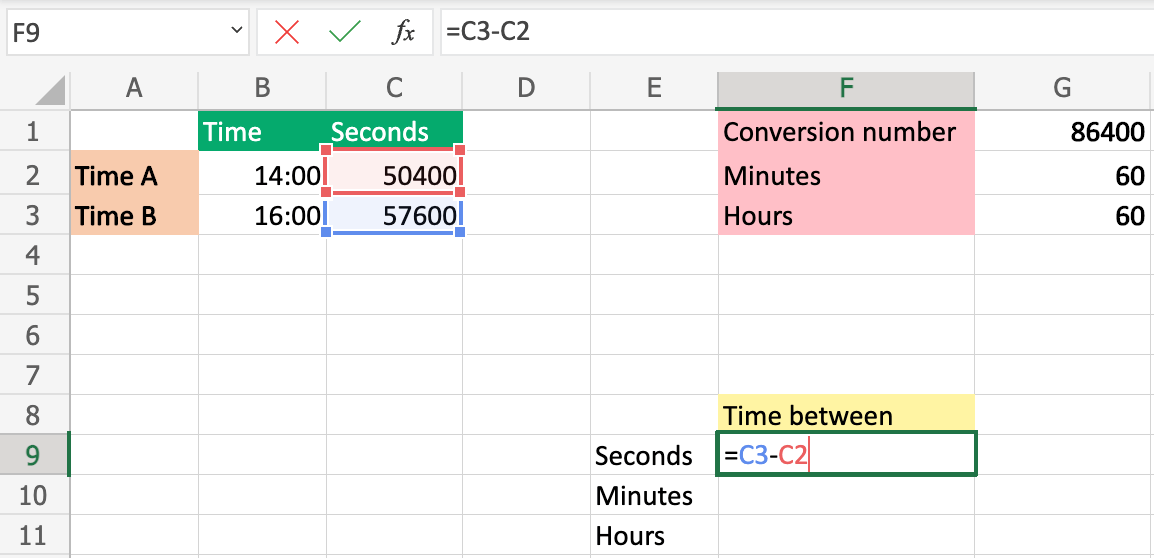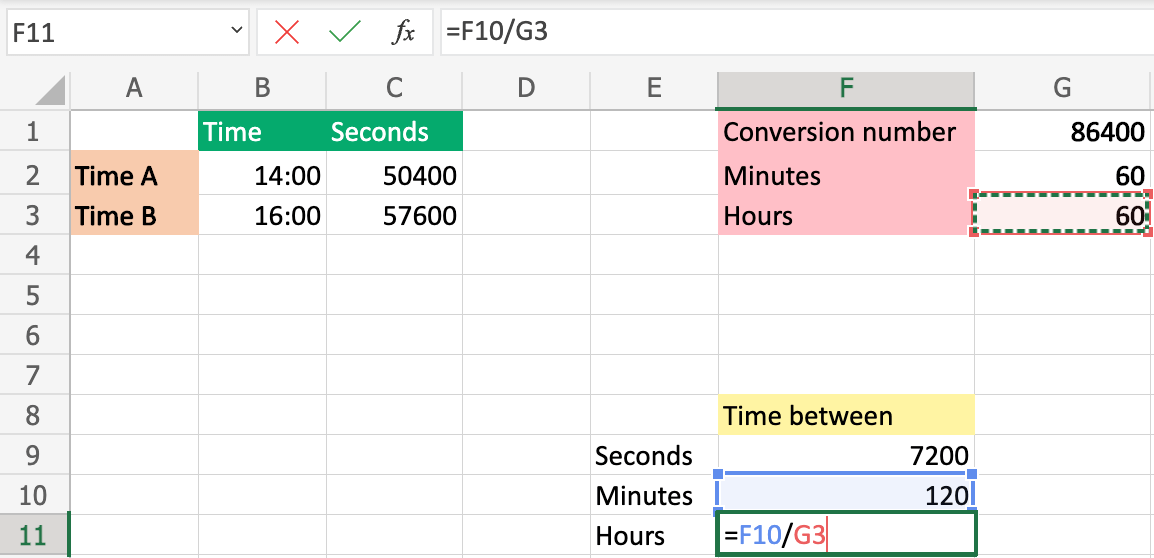# How to Find the Difference Between Two Times Using Excel

Learn how to find the difference between two times using Excel.

Time can be converted into seconds using Excel.

The number `86400` can be used to convert time to seconds.Read more about 86400 in the How to Convert Time to Seconds Chapter

## How to find the difference between two times?

##### Step 1) Create a sheet and set up values:

In this example, we will find the seconds between `14:00(A2)`Time A and `16:00(A3)` Time B.

Copy the values to follow the example.

Paste the values directly into your sheet.Copy Values

Info:

Excel can change the format when you use the 86400 number.

Make sure to change the format back to number if it changes to time format.

##### Step 2) Convert time to seconds:

Let us multiply the time by 86400.

Select cell `C2`

Convert time to seconds for Time A:

### Calculation

` B2(14:00) * G1(86400) = 50400`Repeat the same for Time B: (`B3(16:00) * G1(86400)`)##### Step 3) Find the difference in seconds:

Select cell `F9`

Deduct Time B from Time A to find the difference.

Use the converted seconds to calculate (the numbers in Column C).

### Calculation

` C3(57600) - C2(50400) = 7200 Seconds`##### Step 4) Convert Seconds to Minutes:

Divide the seconds on 60 to find the minutes.

Select cell `F10`

### Calculation

` F9(7200) / G2(60) = 120 Minutes`##### Step 5) Convert Minutes to Hours:

Divide the minutes on 60 to find the hours.

Select cell `F11`

### Calculation

` F9(120) / G3(60) = 2 Hours`Good job!

You have successfully found the time between two times, `14:00` and `16:00`.

In this case, the right answer was `7200 Seconds or 120 Minutes or 2 hours`.

W3Schools is optimized for learning and training. Examples might be simplified to improve reading and learning. Tutorials, references, and examples are constantly reviewed to avoid errors, but we cannot warrant full correctness of all content. While using W3Schools, you agree to have read and accepted our terms of use, cookie and privacy policy.